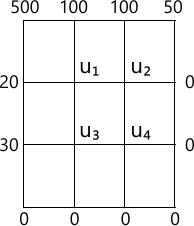MORE IN Engineering Mathematics 3
VTU Electronics and Communication Engineering (Semester 3)
Engineering Mathematics 3
December 2013
Total marks: --
Total time: --
INSTRUCTIONS
(1) Assume appropriate data and state your reasons
(2) Marks are given to the right of every question
(3) Draw neat diagrams wherever necessary

1 (a) Find the Fourier series expansion of the function f(x)=|x| in (-Π, Π), hence deduce that $\dfrac {\pi^2}{8} = \sum^{\infty}_{n=1} \dfrac {1}{(2n-1)^2}$
6 M
1 (b) Obtain the halft-range cosine series for the function;, f(x)=(x-1)2 in the interval 0?x?1 and hence show that $\pi^2 = 8 \left \{ \dfrac {1}{1^2}+ \dfrac {1}{3^2}+ \dfrac {1}{5^2}+ \cdots \ \cdots \right \}$
7 M
1 (c) Compute the constant term and first two harmonics of the Fourier series of f(x) given by.
 x 0 Π/3 2Π/3 Π 4Π/3 5Π/3 2Π f(x) 1 1.4 1.9 1.7 1.5 1.2 1.0
7 M

2 (a) Obtain the Fourier cosine transform of $f(x)= \dfrac {1}{f+x^2}$
6 M
2 (b) Find the Fourier transform of $f(x) = \left\{\begin{matrix} 1-x^2 &for \ |x| \le 1 \\ 0 & for \ |x| > 1 \end{matrix}\right. \ and \ evaluate \ \int_c \dfrac {x \cos x - \sin x}{x^3}dx$
7 M
2 (c) Find the inverse Fourier sine transform of $\dfrac {s}{1+s^2}$
7 M

3 (a) Obtain the various possible solution of two dimensional Laplace's equation, uxx+uyy=0 by the method of separation of variables.
7 M
3 (b) Solve the one-dimensional wave equation $C^2 \dfrac {\partial^2 u}{\partial x^2} = \dfrac {\partial^2 u}{\partial t^2}, \ 0 7 M 3 (c) Obtain the D'Alembert's solution of the wave equation un=C2 uxx subject to the conditions \[ u(x,0)=f(x) = \ and \ \dfrac {\partial u}{\partial t} (x, 0) = 0$
6 M

4 (a) Find the best values of a,b,c if the equation y=a+bx+cx2 is to fit most closely to the following observations.
7 M
4 (b) Solve the following by graphical method to maximize z=50x+60y subject to the constraints. 2x+3y?1500. 3x+2y?1500. 0?x?400 and 0?y?400.
6 M
4 (c) By using Simplex method. Maximum P=4x1-2x2-x3 subject to the constraints, $x_1 + x_2+x_3 \le 3 , \ 2x_1+2x_2+x_3 \le 4, \ x_1-x_2\le 0. x_1 \ge 0 \ and \ x_2 \ge 0.$
7 M

5 (a) Using Newton-Raphson method. Find a real root x sin x + cos x =0 nearer to Π carryout three iterations upto 4-decimals places.
7 M
5 (b) Find the largest Eigen value and the corresponding Eigen vector of the matrix. $\begin{bmatrix} 2 &-1 &0 \\-1 &2 &-1 \\ 0 &-1 &2 \end{bmatrix}$ By using the power method by taking the initial vector as [ 1 1 1]1 carryout 5-iterations.
7 M
5 (c) Solve the following system of equations by Relaxation method:
12x+y=z=31; 2x+8y-z=24; 3x+4y-10z=58
6 M

6 (a) A survey conducted in a slum locality reveals the following information as classified below.
\n \n \n \n \n \n \n \n \n \n \n \n \n \n \n \n \n \n \n
 \n Income per day in\n Rupees 'X' \n Under 10 \n 10 - 20 \n 20 - 30 \n 30 - 40 \n 40 - 50 \n Number of\n Persons 'y' \n 20 \n 45 \n 115 \n 210 \n 115

Estimate the probable number of persons in the income group 20 to 25.
7 M
6 (b) Determine f(x) as a polynomials in x for the data given below by using the Newton's divided difference formula.
 x 2 4 5 6 8 10 f(x) 10 96 196 350 868 1746
7 M
6 (c) Evaluate $\int^1_c \dfrac {x}{1-x} dx$ by using Simpson's $\left (\dfrac {1}{3} \right )^{nd}$ rule by taking 6- equal strips and hence deduce an approximate value of logc2.
6 M

7 (a) Solve the wave equation $\dfrac {\partial^2 u}{\partial t^2} = 4 \dfrac{\partial ^2 u}{\partial x^2}$ subject to u(0,1)=0. U(4, t)=0, u1(x,0)=0 and u(x,0)=x(4-x) by taking h=1, K=0.5 upto 4-steps.
7 M
7 (b) Solve numerically the equation $\dfrac {\partial u}{\partial t} = \dfrac {\partial^2 u} {\partial x^2}$ subject to the conditions, u(0,t)=0 = u (1, t). t?0 and u(x,0)=sin Πx, 0?x?1. Carryout the computation for two levels taking h=1/3 and K=1/36.
7 M
7 (c) Solve uxx+uyy=0 in the following square region with the boundary conditions as indicated in the Fig. Q7 (c)6 M

8 (a) Find the z-transform of. (i) sinh nθ (ii) cosh nθ (iii) n2.
7 M
8 (b) Find the inverse z-transform of $\dfrac {2z^2 + 3z}{(z+2)(z-4)}$
6 M
8 (c) Solve the difference equation yn+2+6yn+1+9yn=2n with y0=y1=0 by using z-transform.
7 M

More question papers from Engineering Mathematics 3